# Supervised, Unsupervised, And Semi-Supervised Learning With Real-Life Usecase

In our previous article (here), we discussed the classification of the Machine Learning models on five different bases. Based on the nature of input data that we provide to the machine learning algorithms, machine learning can be classified into 4 major categories.

1. Supervised Learning
2. Unsupervised Learning
3. Semi-Supervised Learning
4. Reinforcement Learning

### Key questions that are being answered in this blog :

1. What are Supervised, unsupervised, semi-supervised, Reinforcement Learning, and how are they related to each other?
2. How classification, regression, or clustering algorithms are linked with supervised and unsupervised learning?
3. Why these terms named "Supervised" and "Unsupervised"?
4. What are the famous algorithms associated with supervised or unsupervised approaches?
5. Why most of the real-life scenarios are more likely to be related to semi-supervised learning?

### Supervised Learning

Supervised Learning is a category in which we feed labeled data as input to the machine learning model.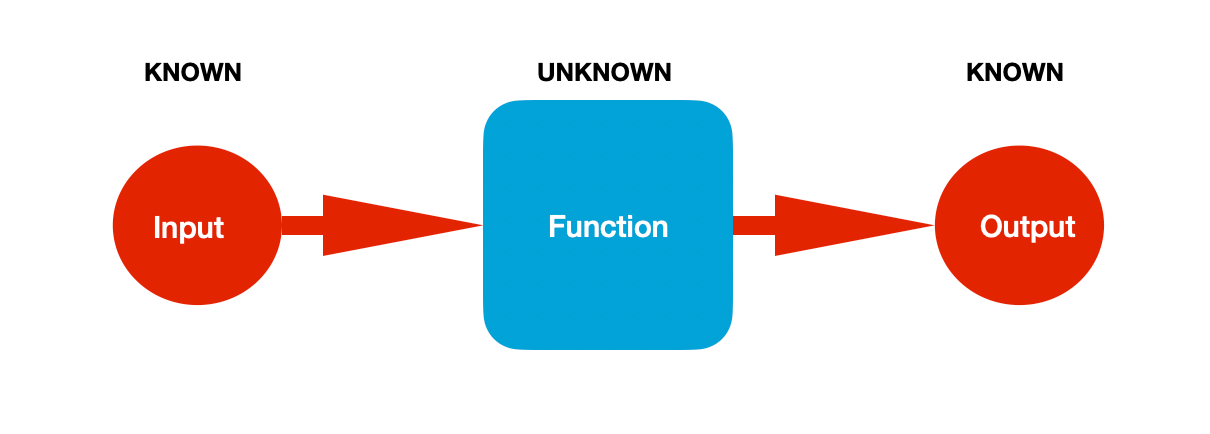The input and output values are already known, and the machine learning algorithm learns the mapping function. Mathematically, for Y as the Output and X as the input, machine learning algorithms try to find the best mapping function f such that Y = f(X).

If you observe closely, learning happens like some supervisor is supervising the process of the learning. We already know the answers; hence algorithms try to map the function so that the predicted answers must be close to the actual answers. Let’s say the machine has learned a mapping function f predicting the values Y’ for every X. Once the difference between predicted (Y’) and actual (Y) goes below a certain threshold, and it means model performance is not improving further and hence learning stops.

#### Supervised learning can be further categorized as

1. Classification:- Taking the example of the below image, images of shapes are the input to the machine learning model, and labeling of those images as shape names ( This process is called annotation ) is the output data. Based on these input and output data, the model learns to predict the category of unseen image data, whether it is a rectangle, circle, triangle, or hexagon.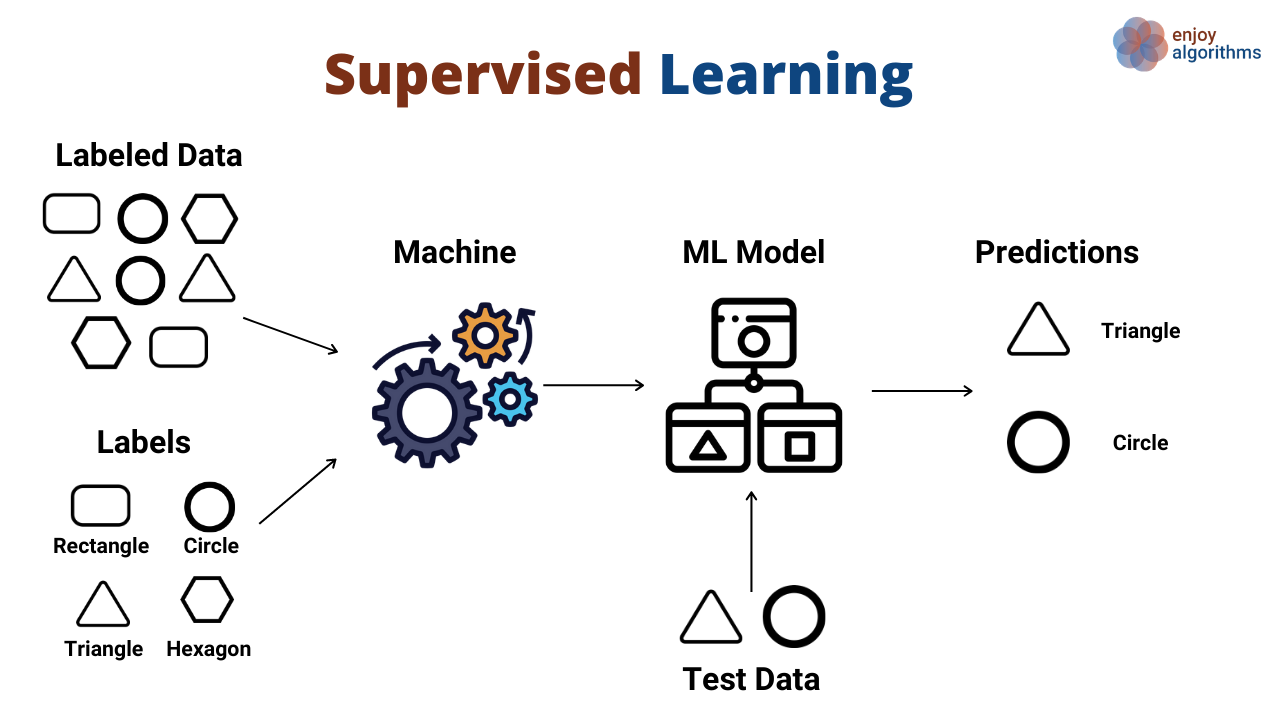2. Regression:- Taking the example of the below image, there is the experience (in years) on the X-axis. For every experience, there is one salary (in per month Rupees) on the Y-axis. Green dots are the coordinates (X, Y) in the form of Input and Output data. The regression problem tries to find the continuous mapping function from input to output variables.

In the below image, if the order of the mapping function is fixed to 1, which is a linear function, the model will learn the black line shown in the image.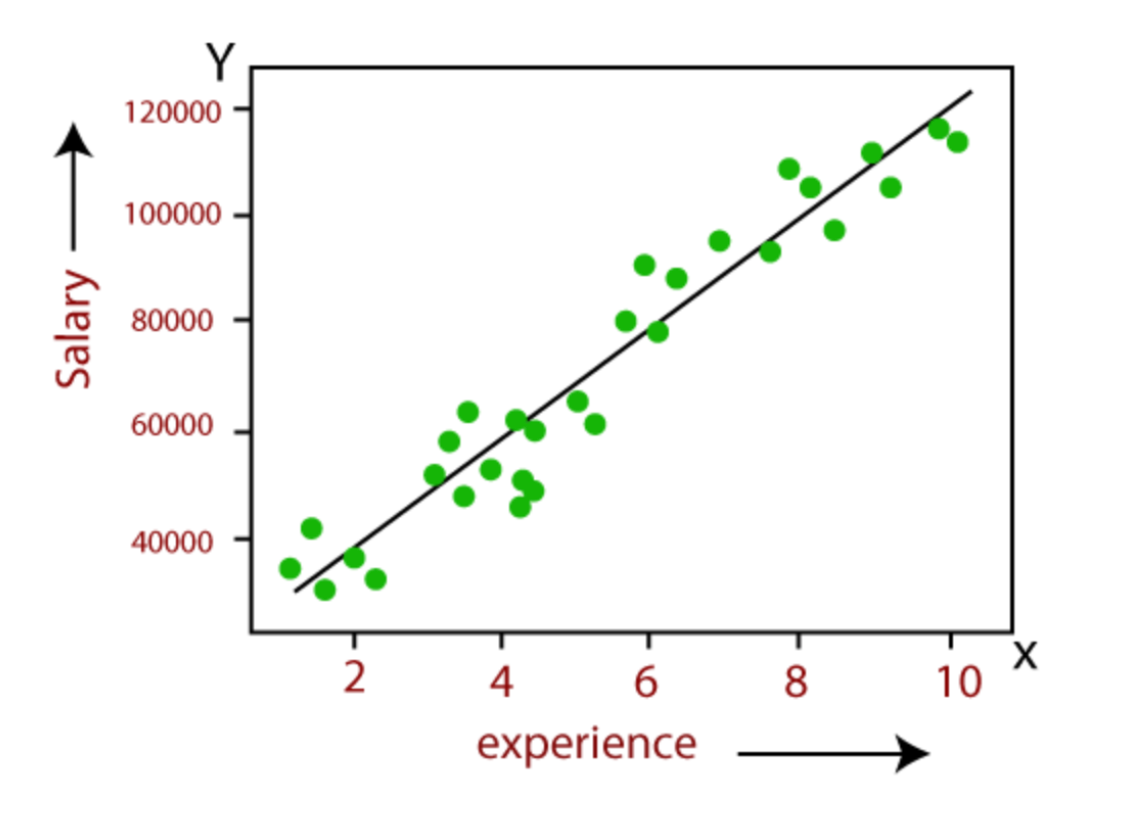Credit: Websystemer

#### Some famous use cases of Supervised Learning are :

• Object detection and Image Classification.
• Recommendation systems.
• Time Series prediction.

#### Some Frequently used algorithms in Supervised Learning :

• Linear & Logistic Regression
• SVM (Support Vector Machines)
• Random Forest

### Unsupervised Learning

Unsupervised learning is a category of machine learning in which we only have the input data to feed to the model but no corresponding output data.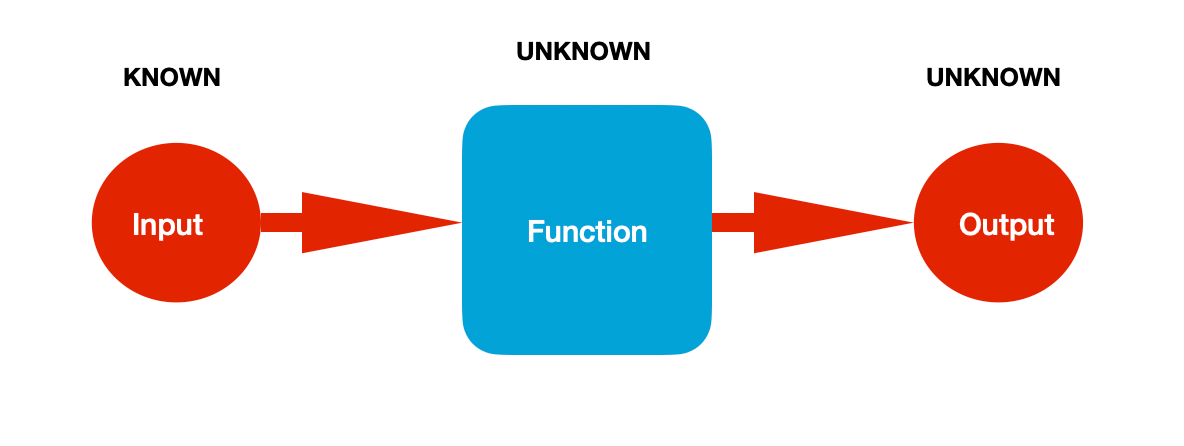Here, we know the value of input data, but the output and the mapping function both are unknown. In such scenarios, machine learning algorithms find the function that finds similarity among different input data instances and groups them based on the similarity index, which is the output of unsupervised learning.

In such learning, there is no supervision as there is no existence of output data. Hence they are called Unsupervised learning. Algorithms try to find the similarity between different instances of input data by themselves using a defined similarity index.

Unsupervised Learning can further be categorized as :

1. Clustering (Unsupervised classification):- Taking the example of the below image, we have input data consisting of images of different shapes. Machine learning algorithms try to find the similarity among different images based on the color pixel values, size, and shapes and form the groups as outputs in which similar input instances lie.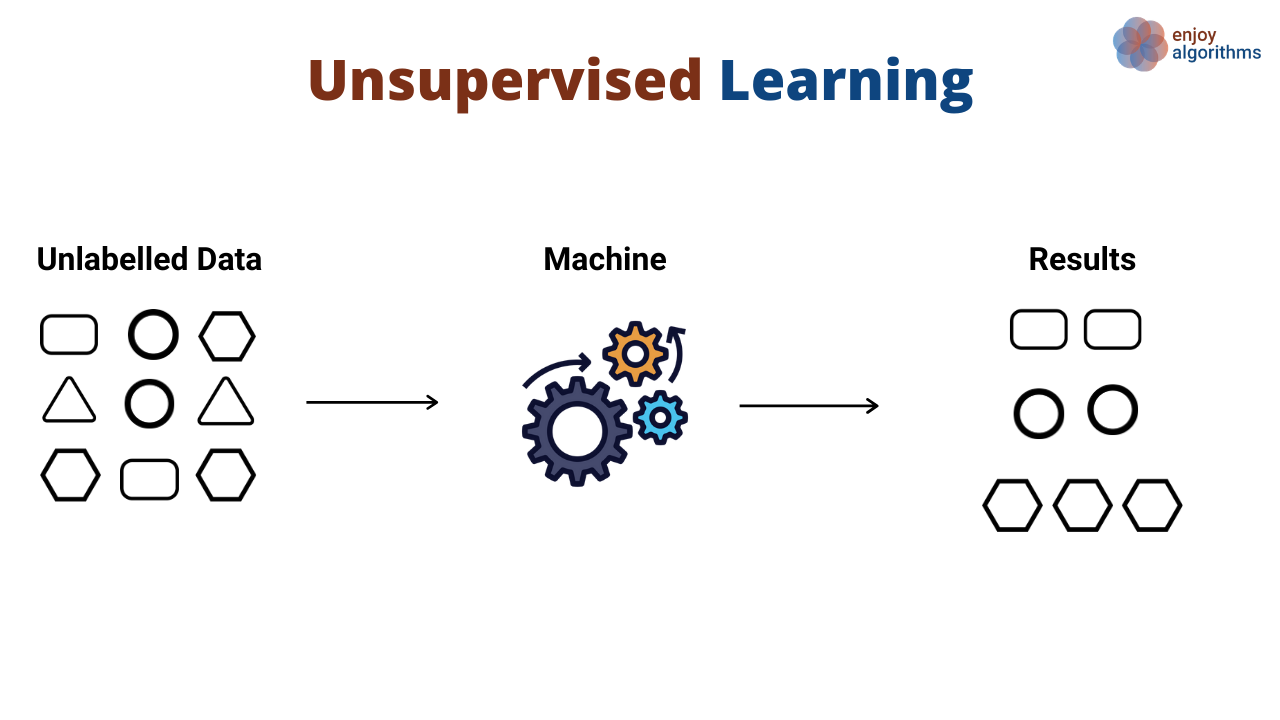##### Clustering Algorithms are :
• Hierarchical clustering
• K-means clustering

2. Dimensionality Reduction:- When the attributes of the data samples have more than three dimensions, there is no way to visualize the relationship among the attributes as we can not plot variables in more than 3 Dimensions. But without analyzing the input data, we can never be sure about the Machine Learning model's performance. To solve this purpose, there are dimensionality reduction techniques using which we bring down the total number of dimensions and analyze the data.

3. Association:- Taking the example of the below image, such learning is more about discovering rules that describe a large portion of the data. Customers who bought a banana also bought carrots, or Customers who bought a new house also bought new furniture.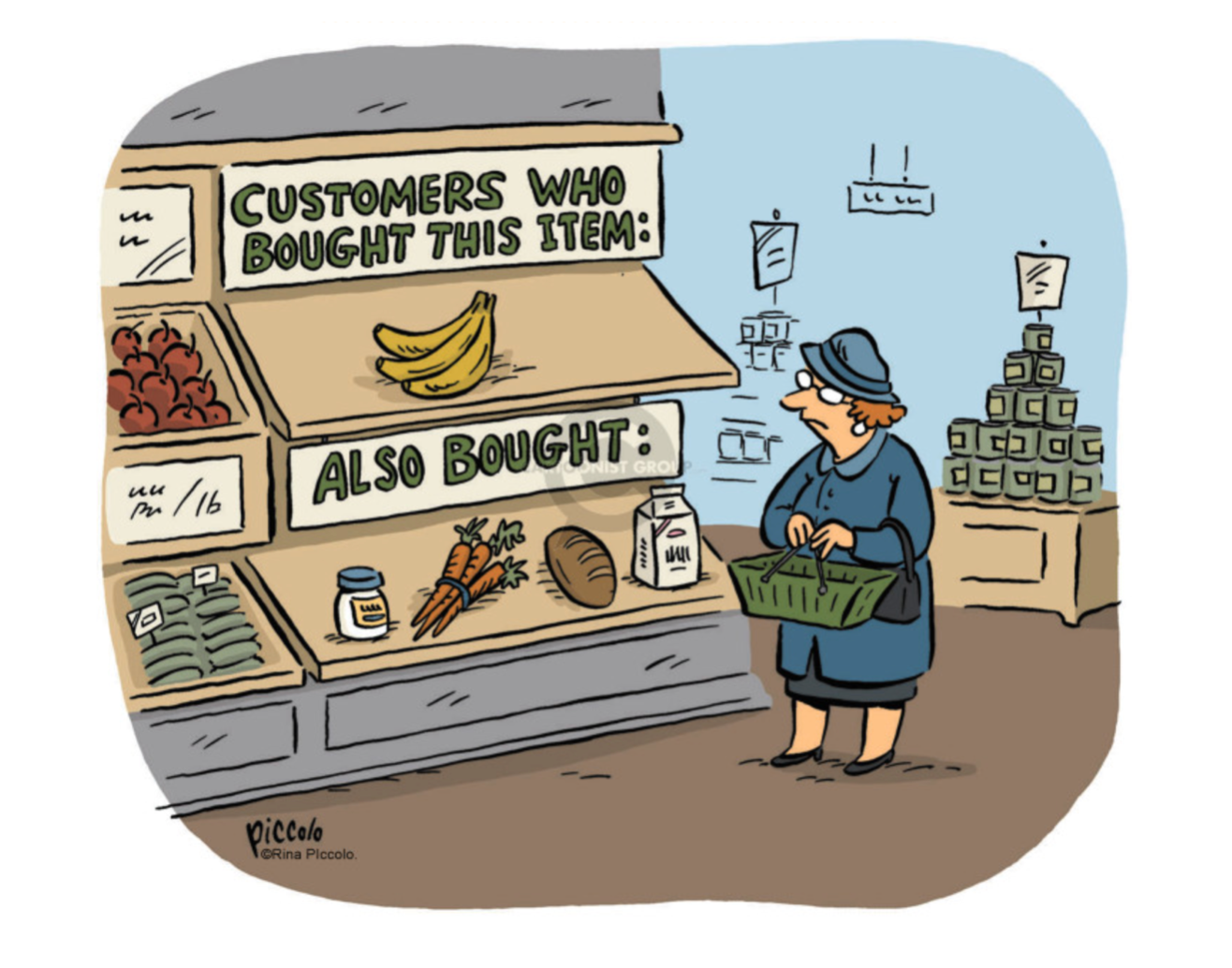Credit: Cartoonistgroup

#### Some famous use cases of Unsupervised Learning are :

1. Market Segmentation
2. Fraud detection
3. Image Segmentation

#### Some Frequently used algorithms in Unsupervised Learning :

1. K-means
2. Apriori Algorithm for learning association rule.
3. Principal Component Analysis

### Semi-Supervised Learning

Semi-supervised learning is a category of machine learning in which we have input data, and only some of those input data are labeled as the output.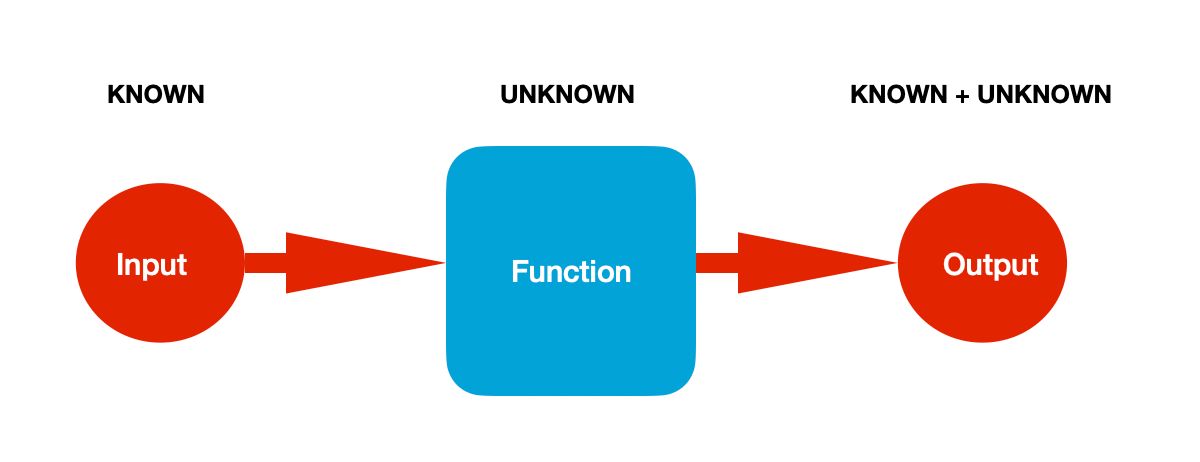Semi-supervised learning is partially supervised and partially unsupervised.

Let’s take one example from the below image to make it clear, suppose there is a bucket consisting of three fruits, apple, banana, and orange. Someone captured the image of all three but labeled only orange and banana images.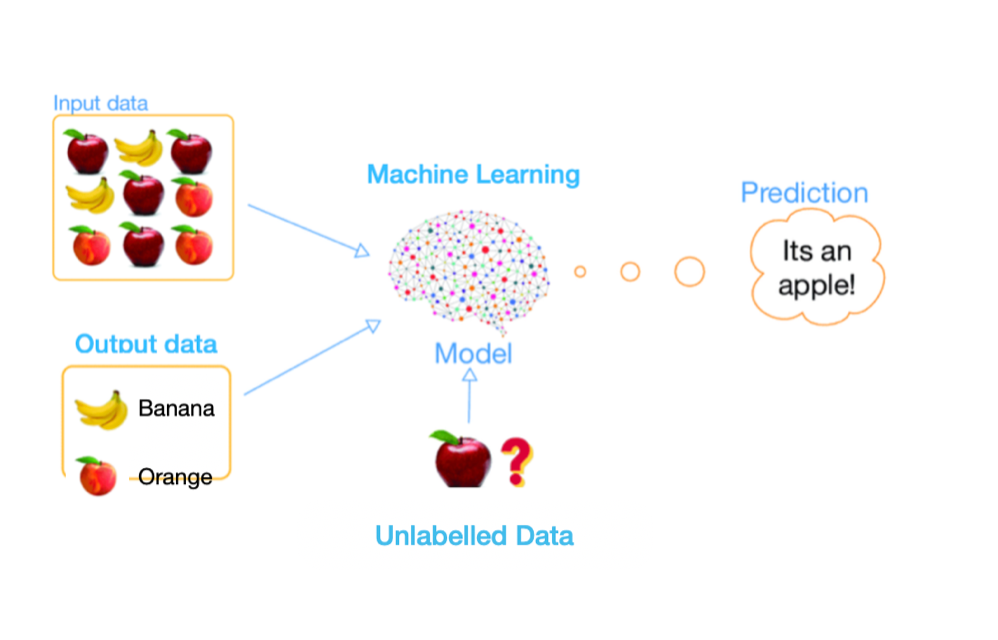Nowadays, it has become a trend to capture a tremendous amount of data. Many big companies have already collected millions of Terrabytes of data and are still collecting. But labeling the collected data requires workforce and resources, and hence it's too expensive. And this is the main reason that many real-life databases fall in this category.

#### Some famous use cases of Semi-supervised Learning are :

In such type of learning, one can use either.

• Supervised learning on unlabeled data and use the predicted output as input for retraining other supervised learning models and test it on other unlabeled data.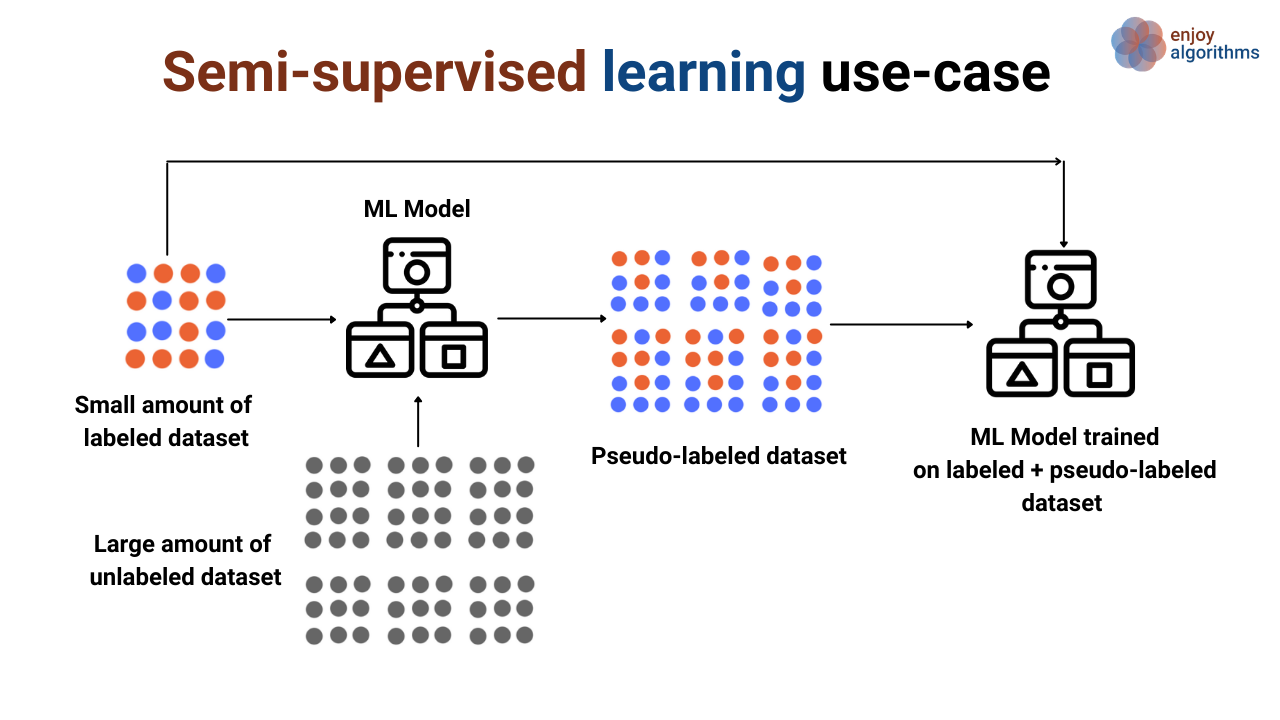For example, suppose there is a large chunk of data in the image above, and a small amount of labeled dataset is present. We can train the model using that small amount of labeled data and then predict on the unlabelled dataset. Prediction on an unlabelled dataset will attach the label with every data sample with little accuracy, termed as a Pseudo-labeled dataset. Now a new model can be trained with the mixture of the true-labeled dataset and pseudo-labeled dataset.

Or

• Unsupervised learning to capture and learn the structure present in the data.

### Reinforcement Learning

Here, ML algorithms act as agents in the environment where these agents choose the possible options of actions. The agent selects the best action from all the options present at that environmental state, and based on that selection, receives reward/risks. The algorithms keep an eye on maximizing the reward and reduce the risk, and eventually learns.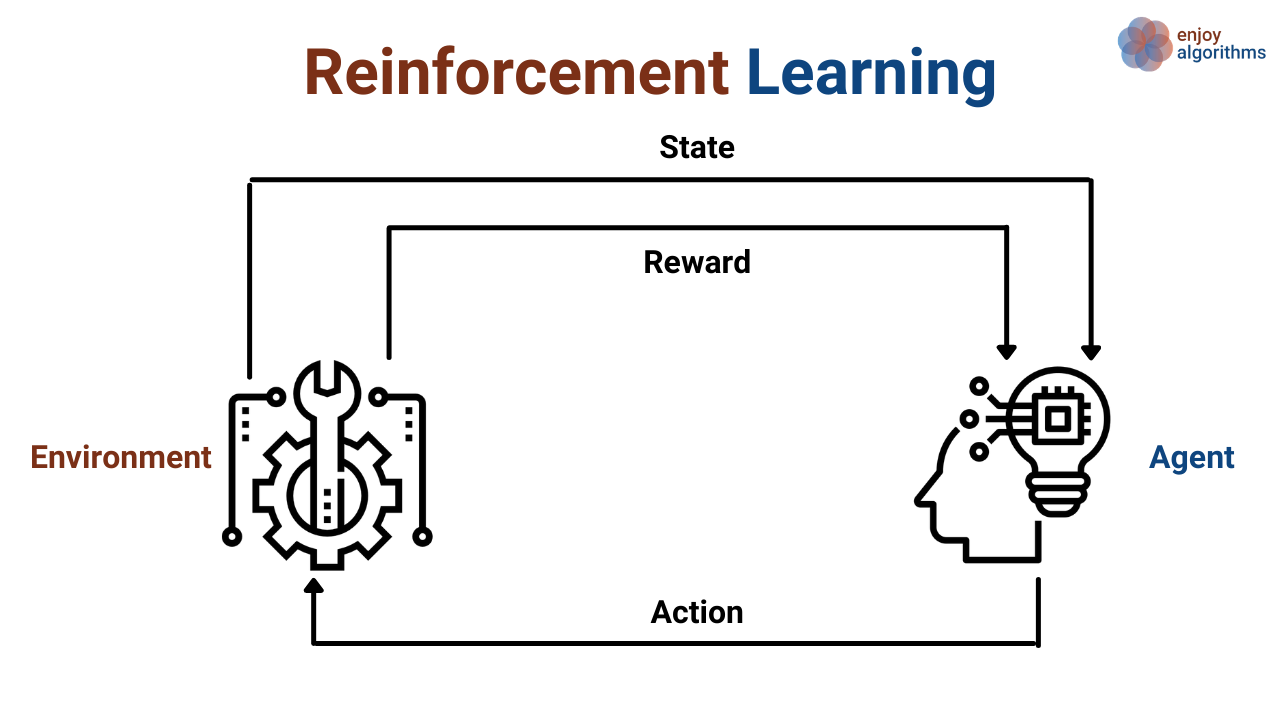#### Some famous use cases of Reinforcement Learning are :

If we look at the history of machine learning, we will find that RL is quite old and in the industry for a longer period of time. But because of the requirement of awareness of the complete environment, it is usually used with simulated environments. Some of the most common use-cases where it is being used in the industry are,

1. The agent that can drive the vehicle inside the simulated environment.
2. Predicting the stock price in the stock market.
3. Agents that can play games.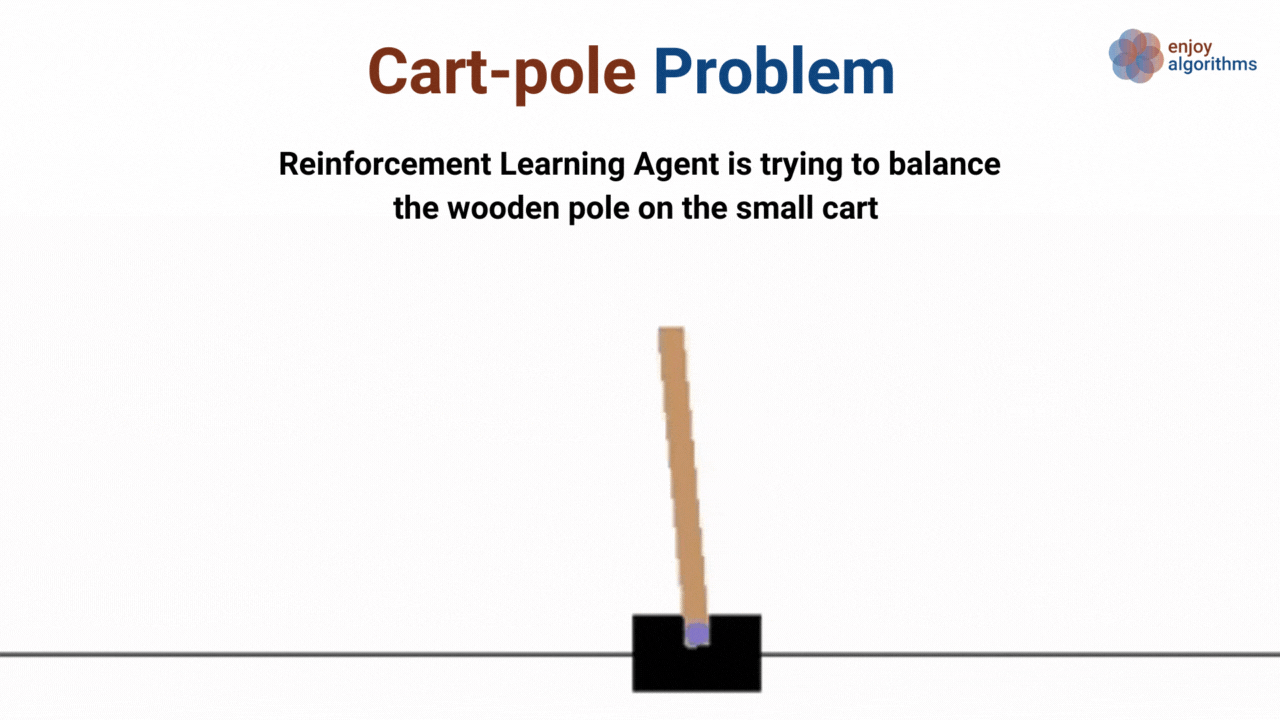#### Some Frequently used algorithms in Reinforcement Learning :

1. Q-Learning
2. Deep Q-Learning (DQN)

### Critical Questions to explore

1. Why we name different machine learning algorithms as supervised or unsupervised learning?
2. Why is semi-supervised learning the most common case in Machine Learning?
3. How can we utilize semi-supervised learning in case of object detection problems?
4. Can we think of a reason why supervised learning can never bring that future what we are expecting from Machine Learning?
5. Why is the annotation process required?

### Conclusion

In this article, we described machine learning classification based on the “Nature of input data.” We came across the definition of Supervised, Unsupervised, Semi-Supervised, and Reinforcement Learning and talked about some industry use-case or real-life use-case of these categories. We also listed some famous algorithms associated with each category. We hope you have enjoyed the article.

### Quick Note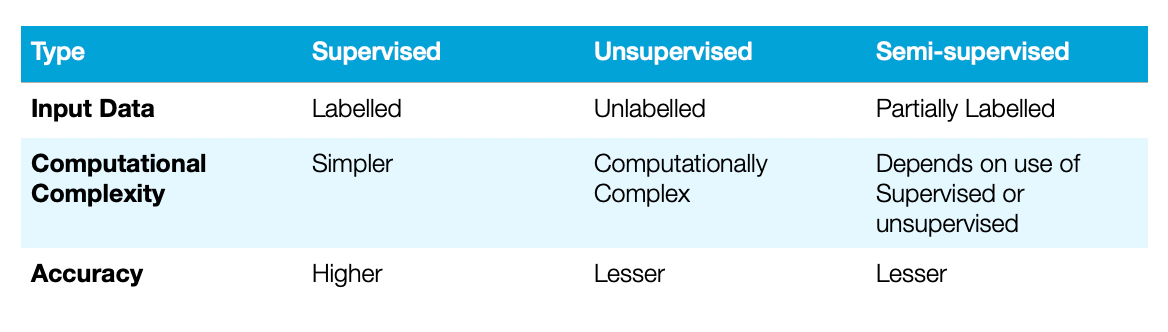### Enjoy Learning! Enjoy Thinking! Enjoy Algorithms!

Get well-designed application and interview centirc content on ds-algorithms, machine learning, system design and oops. Content will be delivered weekly.